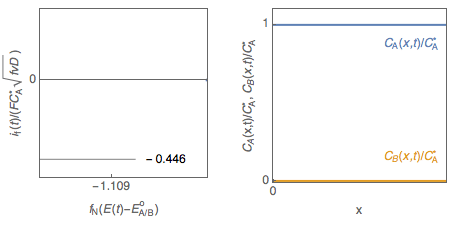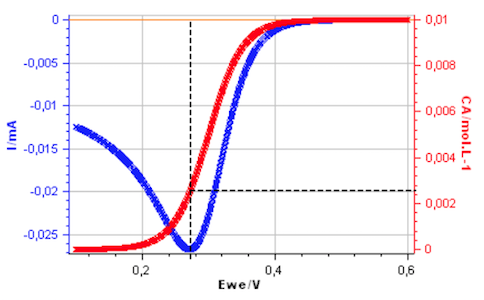Topic 5 min read# CVSim as a learning tool. II Interfacial concentrations

Latest updated: March 25, 2022

## Cyclic voltammetry and interfacial concentrations of electroactive species

Let us consider a reversible, or Nernstian, redox reaction (E) A + e $\leftrightarrow$ B assuming semi-infinite linear diffusion and a solution initially containing only species A ($C_\mathrm B^*=0$), studied by cyclic voltammetry. The change of the concentrations of species A and B with electrode potential is shown in Fig. 1. It is tempting to explain qualitatively the shape of the voltammogram by saying that the interfacial concentration in A decreases and becomes zero at the peak potential, where the current is maximum in absolute value.Figure 1: Cyclic voltammetry for reversible redox reaction and change of the concentration profiles of species A and B with electrode potential.

However, the interfacial concentration $C_\mathrm A(0,t)$ is not close to zero at the peak potential as shown in Fig. 2, plotted using CVSim.

## Interfacial concentration of A at the current peak

The potential for which the current reaches an extremum, also named peak potential $E_{\mathrm{p}}$ is given for equal diffusion coefficients of the two species A and B by [1,2]

$$E_{\mathrm{p}}=E^\mathrm o_\text{A/B} -\frac{1,109}{f_\mathrm N} \Rightarrow f_\mathrm N(E_{\mathrm{p}}-E^\mathrm o_\text{A/B})=-1,109,\;f_\mathrm N=F/(R\,T) \tag 1$$

For a Nernstian redox reaction the interfacial concentrations $C_\mathrm A(0,t)$ and $C_\mathrm B(0,t)$ of species A and B follow Nernst’s law outside equilibrium

$$E(t)=E^\mathrm o_\text{A/B}+\frac{1}{f_\mathrm N}\,\ln \frac{C_\mathrm A(0,t)}{C_\mathrm B(0,t)}\Rightarrow f_\mathrm N(E(t)-E^\mathrm o_\text{A/B})=\ln \frac{C_\mathrm A(0,t)}{C_\mathrm B(0,t)} \tag 2$$ and, for the peak potential $t=t_\mathrm{p}$Figure 2: Cyclic voltammetry for a reversible redox reaction and change of $C_\mathrm A(0,t)$ with electrode potential.

## Interfacial concentration of A at the current peak

The potential for which the current reaches an extremum, also named peak potential $E_{\mathrm{p}}$ is given for equal diffusion coefficients of the two species A and B by [1,2]

$$E_{\mathrm{p}}=E^\mathrm o_\text{A/B} -\frac{1,109}{f_\mathrm N} \Rightarrow f_\mathrm N(E_{\mathrm{p}}-E^\mathrm o_\text{A/B})=-1,109,\;f_\mathrm N=F/(R\,T) \tag 1$$

For a Nernstian redox reaction the interfacial concentrations $C_\mathrm A(0,t)$ and $C_\mathrm B(0,t)$ of species A and B follow Nernst’s law outside equilibrium

$$E(t)=E^\mathrm o_\text{A/B}+\frac{1}{f_\mathrm N}\,\ln \frac{C_\mathrm A(0,t)}{C_\mathrm B(0,t)}\Rightarrow f_\mathrm N(E(t)-E^\mathrm o_\text{A/B})=\ln \frac{C_\mathrm A(0,t)}{C_\mathrm B(0,t)} \tag 2$$

and, for the peak potential $t=t_\mathrm{p}$

$$\frac{C_\mathrm A(0,t_{\mathrm{p}})}{ C_\mathrm B(0,t_{\mathrm{p}})}=\exp(-1,109) \tag 3$$

Writing, as in the steady state conditions, that the concentrations $C_\mathrm A(0,t)$ and $C_\mathrm B(0,t)$ are related, for equal diffusion coefficients of the two species A and B, by the relationship

$$C_\mathrm A(0,t_{\mathrm{p}})+C_\mathrm B(0,t_{\mathrm{p}})=C_\mathrm A^* \tag 4$$

since $C_\mathrm B^*=0$.

Using the Eqs.~(3) et (4), we have:

$$\frac{C_\mathrm A(0,t_{\mathrm{p}})}{C_\mathrm A^*}= 24.8\;\% \tag 5$$

The interfacial concentration of A, for a Nernstian redox reaction, is therefore not close to zero at the peak potential of the voltammogram in agreement with Fig. 2.

 A. J. Bard and L. R. Faulkner. Electrochemical methods. Fundamentals and Applications. John Willey & Sons, New York, 1980.

 J.-P. Diard, B. Le Gorrec, and C. Montella. Cinétique electrochimique. Hermann, Paris, 1996.

CVSim cyclic voltammetry interfacial concentrations peak current